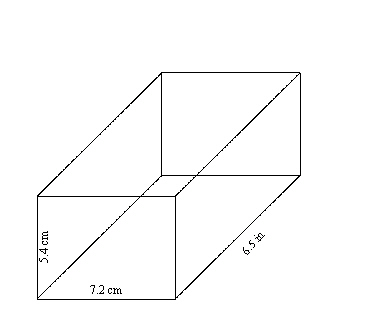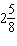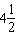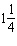Name:    Math 10W Unit 3 Practice Test #3

Multiple Choice
Identify the choice that best completes the statement or answers the question.

1.

How many inches is 7'4"?
 a. 55" c. 132" b. 86" d. 88"

2.

What is the circumference of a circular hot tub if its radius is 1.35 m?
 a. 5.72 m c. 12.03 m b. 8.48 m d. 4.24 m

3.

The driving distance from Yellowknife, NWT, to Whitehorse, YT, is 2704 km. What is this distance in miles?
 a. 1352 miles c. 4326 miles b. 1690 miles d. 901 miles

4.

What is the surface area of a cube that measures 16" on each side?
 a. 2048 in2 c. 1024 in2 b. 1536 in2 d. 256 in2

5.

Two cylinders of radius 7 cm are placed together end to end. If the first cylinder was 13 cm long and the second was 8 cm long, what is the surface area of this new cylinder?
 a. 1477.8 cm2 c. 1231.5 cm2 b. 1539.4 cm2 d. 985.2 cm2

6.

Volume is measured in which type of units?
 a. linear units c. cube units b. square units d. zero units

7.

How many mL are in 1 cup?
 a. 100 mL c. 300 mL b. 250 mL d. 500 mL

8.

Find the volume of the rectangular prism below, in cm3.a. 708.5 cm3 c. 493.6 cm3 b. 252.7 cm3 d. 641.5 cm3

9.

How many pints are in 13.9 quarts?
 a. 29.8 pints c. 55.6 pints b. 7.0 pints d. 27.8 pints

10.

A cube has a volume of 1 cubic foot. What is its volume in cubic inches?
 a. 144 cu in c. 1728 cu in b. 1827 cu in d. 168 cu in

1.

Yuna is replacing some plumbing pipes. She needs 3 sections of pipe: one that is' long, one that is' long, and one that is' long. How much pipe does she need if she loses" every time she makes a cut? She can only purchase the pipe in full feet.

2.

A local craft store sells ribbon for \$1.37/m. The pattern for Marnie’s Halloween costume requires 4 feet of ribbon. How much will it cost Marnie to buy the ribbon?

3.

Michael has been training to run a 4-minute mile. He manages to run 1700 m in 4 minutes. Did he succeed?

4.

The circumference of a circular fountain is 30 inches.

a)       What is the circumference in centimetres, to one decimal place?

b)       What is the radius of the fountain in centimetres, to one decimal place?

5.

A garden measures 14 yd by 10 yd. In order to grow well, vegetables need about 0.8 m2 of space each. How many plants can you expect to grow in the garden?